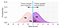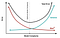# Performance Metrics for Classification Models in Machine Learning: Part II

## Evaluate Multi-Class Classification Models

In part I, we discussed how to evaluate binary-class classification models using Recall, Precession, Accuracy, and F1-Score. Here, we will see how we can apply those metrics to a multi-class classification model.

As seen in part I, we can build the confusion matrix for a multi-class model as well as the binary-class model. But as it may become more complex when there are too many classes, we can separate each class in a single confusion matrix to make calculations and visualizations easier.

Note: Of course we will not do that manually for each classification problem we work with, but this…

# Performance Metrics for Classification Models in Machine Learning: Part I

## Evaluation Metrics for Binary-Class Classification ModelsSource: https://lukeoakdenrayner.files.wordpress.com/2018/01/threshold2.png

After building a machine learning model and before deploying, the model should be evaluated and tested to make sure it does its job correctly and meets the desired objectives. In this blog post, we are going to talk about some of the evaluation metrics that are used for classification models and how to use them for binary classification. Today we will talk about:

• Accuracy
• Recall
• Precision
• F-Beta Score
• AUC/ROC

Before going deep, we need first to learn about what is called the Confusion Matrix. The confusion matrix is simply a visual representation of the model performance:

# Regularization: A Method to Solve Overfitting in Machine Learning

## Know about regularization, what it is, its types and how it can reduce variance and solve overfitting.

Prerequisites:

• Before going on this article, make sure you have a good knowledge about bias and variance in context of machine learning and also know about overfitting. If not, please check this article first.
• You also need to be familiar with cost function and gradient descent. If not, read about them here.

As you remember in our previous article Bias and Variance, one of our models had a low bias and a high variance. We called that overfitting as the regression line perfectly fitted the training data and it failed to fit or even give good predictions for the testing…

# Bias and Variance

## Overview on Bias and Variance in Machine LearningSource: http://scott.fortmann-roe.com/docs/BiasVariance.html

If you are familiar with Machine Learning, you may heard about bias and variance. But if not, don’t worry, we’re going to explain them in a simple way step-by-step.

Let’s use a reverse approach, we will start with a practical example and walk through until we reach the final definition.

We are going to use the Longleys Economic Regression dataset from Kaggle. It is a very simple and small dataset which will be suitable to understand our topic today.

Now let’s have a quick look on the dataset.

# Probability vs Likelihood

## Clear explanation for the difference between Probability and Likelihood with a practical example

In a dictionary, you may find that “probability” and “likelihood” are usually synonyms and sometimes are used interchangeably, but from statistics’ point of view they implicitly refer to distinct things. Probability talks about the outcomes(data)/hypothesis, while likelihood talks about the model/evidence. In this article, we are going to show how probability is different from likelihood and also show some examples.

## Probability

Talks about the data/hypothesis. It refers to finding the chance that a particular event occurs given a distribution of the data or some conditions/evidence. …

# Naïve Bayes Algorithm

## What is Naïve Bayes Algorithm?

Naive Bayes is a classification technique that is based on Bayes’ Theorem with an assumption that all the features that predicts the target value are independent of each other. It calculates the probability of each class and then pick the one with the highest probability. It has been successfully used for many purposes, but it works particularly well with natural language processing (NLP) problems.

Bayes’ Theorem describes the probability of an event, based on a prior knowledge of conditions that might be related to that event.

## What makes Naive Bayes a “Naive” algorithm?

Naive Bayes classifier assumes that the features we use to predict the target are…## Bassant Gamal

Machine Learning enthusiast.